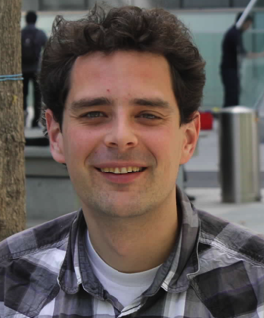# Events

Andreas Wiese: “Approximation algorithms for the Geometric Knapsack problem”
12/OctAbstract: Many optimization problems are NP-hard and therefore we do not expect to find algorithms for them that are efficient, i.e., run in polynomial time, and find the optimal solution for any given instance. Therefore, we are interested in approximation algorithms which are algorithms that run in polynomial time and provably find solutions that differ from the optimum by at most some bounded factor, called the approximation ratio.

In this talk I will present approximation algorithms for the 2-dimensional knapsack problem. Given are a square knapsack and a set of items that are axis-parallel rectangles. Each item has a profit associated with it. The goal is to pack a subset of the given items non-overlappingly into the knapsack in order to maximize the total profit of the packed items. This problem generalizes the well-studied (one-dimensional) knapsack problem. I will present an algorithm with an approximation ratio of 1.89+eps and varios other results for the problem. Key to all results is to show that there are good solutions that have a relatively simple structure.

Bio: Andreas Wiese is an assistant professor at the Industrial Engineering department of the Universidad de Chile. He finished his PhD in 2011 at TU Berlin and was a postdoc at TU Berlin, La Sapienza in Rome and at the MPI for Informatics in Saarbruecken. In his research he focuses on combinatorial optimization and approximation algorithms, i.e., on algorithms that are efficient and compute solutions that are provably close to the optimum.

Fecha: 12 de octubre de 2018, 12.30 a 13.30 horas.

Lugar: Auditorio Phillippe Flajolet, Depto. Ciencias de la Computación, U. de Chile.